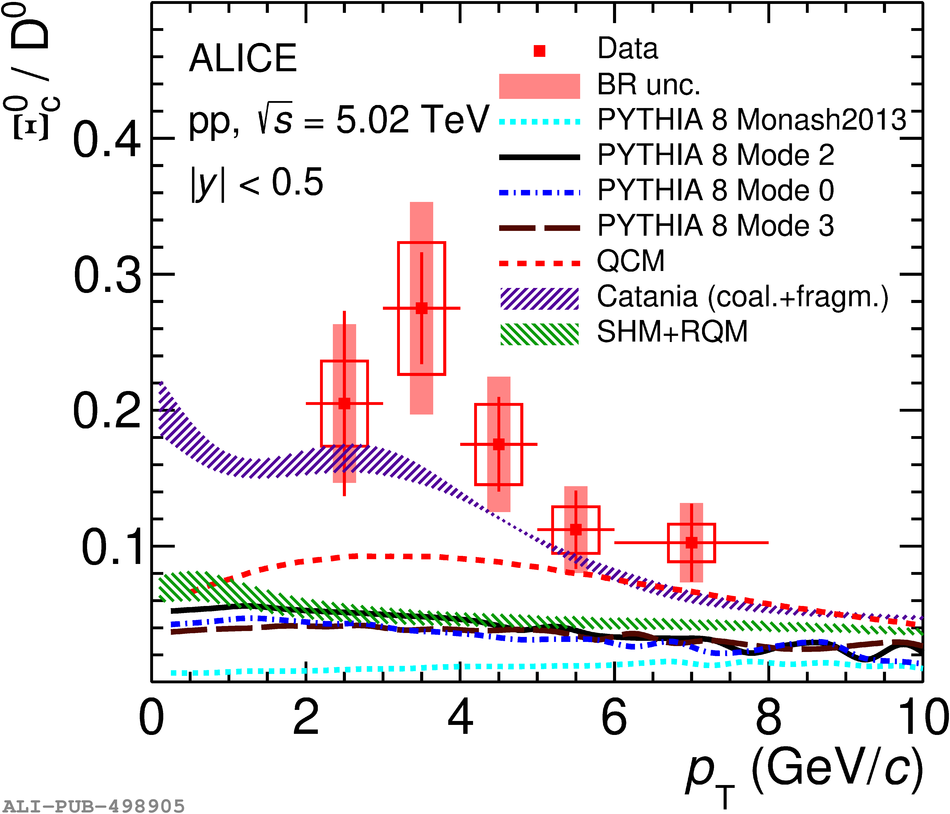Figure 6

 Left panel: \pt-differential production cross section of prompt $\Xi^0_{\rm c}$ baryons in pp collisions at $\sqrt{s}$~=~5.02~TeV compared with model calculations~. Right panel: $\Xi^0_{\rm c}/{\rm D^0}$ ratio as a function of \pt measured in pp collisions at $\sqrt{s}$~=~5.02~TeV compared with model calculations~ (see text for details).